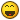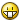# StringSplit use

## Recommended Posts

Not sure why the below code isn't working. I'm looking to split a sting once where the pipe symbol is "|" then split it once more at equals "=" and then write the value to the registry.

```\$TB_1  = "Y"
\$TB_2  = "N"
\$TB_3  = "N"
...
\$TB_31 = "N"

; Toolbar Strings
\$TB_A = "01=New|02=Open|03=metapath|...|31=Print"

For \$i = 1 To 31 Step 1
\$j = Eval( "TB_" & \$i )
\$k = StringSplit( \$TB_A  , '|' )
\$l = StringSplit( \$k[\$i] , '=' )

If \$j = "y" Then
RegWrite  ( \$keyname , \$l , "REG_SZ" , \$l )
EndIf
Next```
##### Share on other sites

I think this will work. I renamed the variables so you know in the future what the variables contain.

Tip:

Use an ini file and loop through the values instead of using: \$TB_1, \$TB_2, etc..

```\$TB_1  = "Y"
\$TB_2  = "N"
\$TB_3  = "N"
...
\$TB_31 = "N"

; Toolbar Strings
\$TB_A = "01=New|02=Open|03=metapath|...|31=Print"

\$toolbar_values = StringSplit(\$TB_A , '|' )

For \$i = 1 To 31 Step 1
\$choice = Eval( "TB_" & \$i )
\$value = StringSplit(\$toolbar_values[\$i] , '=' )
\$value_leftpart = \$value
\$value_rightpart = \$value

If \$choice = "y" Then
RegWrite  ( \$keyname , \$value_leftpart , "REG_SZ" , \$value_rightpart)
EndIf
Next```
##### Share on other sites

Thanks for your time...found the problem tho...not the code...but my folder naming. Hehe its one of those embarassing moments.

```\$g_szVersion = "Notepad2"
If WinExists(\$g_szVersion) Then Exit; It"s already running
AutoItWinSetTitle(\$g_szVersion)```

That was in the script and low and behold I was creating the script in a folder called Notepad2 that was open.Hehe so I'll take my dunce cap and go stand in the corner.Again thanks for the help.## Create an account

Register a new account

• ### Recently Browsing   0 members

×

• Wiki

• Back

• #### Beta

• Git
• FAQ
• Our Picks
×
• Create New...